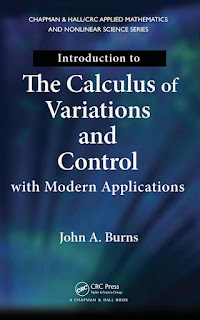CALCULUS MATHEMATICS

# Introduction to the Calculus of Variations and Control with Modern Applications Free DownloadIntroduction to the Calculus of Variations and Control with Modern Applications provides the fundamental background required to develop rigorous necessary conditions that are the starting points for theoretical and numerical approaches to modern variational calculus and control problems. The book also presents some classical sufficient conditions and discusses the importance of distinguishing between the necessary and sufficient conditions. In the first part of the text, the author develops the calculus of variations and provides complete proofs of the main results. He explains how the ideas behind the proofs are essential to the development of modern optimization and control theory. Focusing on optimal control problems, the second part shows how optimal control is a natural extension of the classical calculus of variations to more complex problems.